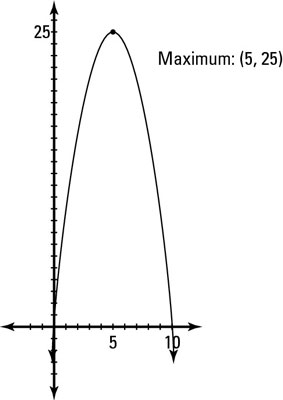##### Pre-Calculus For Dummies, 3rd EditionVertical parabolas give an important piece of information: When the parabola opens up, the vertex is the lowest point on the graph — called the minimum, or min. When the parabola opens down, the vertex is the highest point on the graph — called the maximum, or max.

Only vertical parabolas can have minimum or maximum values, because horizontal parabolas have no limit on how high or how low they can go. Finding the maximum of a parabola can tell you the maximum height of a ball thrown into the air, the maximum area of a rectangle, the minimum value of a company's profit, and so on.

## Finding the max of a parabola

For example, say that a problem asks you to find two numbers whose sum is 10 and whose product is a maximum. You can identify two different equations hidden in this one sentence:

x + y = 10

xy = MAX

If you're like most people, you don't like to mix variables when you don't have to, so you should solve one equation for one variable to substitute into the other one. This process is easiest if you solve the equation that doesn't include min or max at all. So if x + y = 10, you can say y = 10 – x. You can plug this value into the other equation to get the following:

(10 – x)x = MAX

If you distribute the x on the outside, you get 10xx2 = MAX. This result is a quadratic equation for which you need to find the vertex by completing the square (which puts the equation into the form you're used to seeing that identifies the vertex). Finding the vertex by completing the square gives you the maximum value. To do that, follow these steps:

1. Rearrange the terms in descending order.

This step gives you –x2 + 10x = MAX.

2. Factor out the leading term.

You now have –1(x2 – 10x) = MAX.

3. Complete the square.

This step expands the equation to –1(x2 – 10x + 25) = MAX – 25. Notice that –1 in front of the parentheses turned the 25 into –25, which is why you must add –25 to the right side as well.

4. Factor the information inside the parentheses.

This gives you –1(x – 5)2 = MAX – 25.

5. Move the constant to the other side of the equation.

You end up with –1(x – 5)2 + 25 = MAX.

The vertex of the parabola is (5, 25). Therefore, the number you're looking for (x) is 5, and the maximum product is 25. You can plug 5 in for x to get y in either equation: 5 + y = 10, or y = 5.Graphing a parabola to find a maximum value from a word problem
This figure shows the graph of the maximum function to illustrate that the vertex, in this case, is the maximum point.